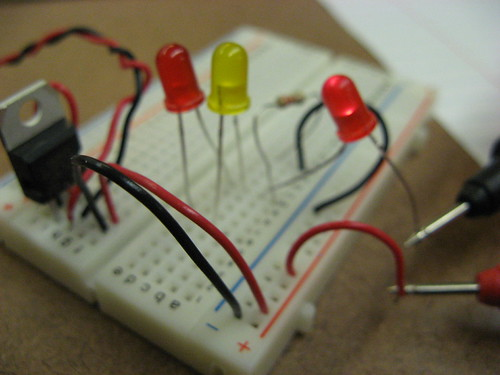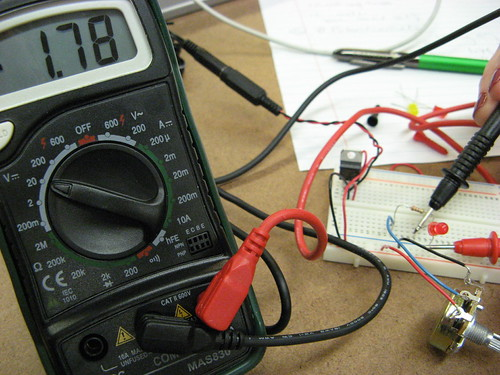# Physical Computing: Week 3 Basic Electronics

I worked with Catherine on this week’s lab in Physical Computing, which involved getting familiar with basic electronics.

First we made a basic LED circuit:

We wired everything up according to the diagram, but nothing happened.  After getting some help from Michael, one of the residents, we discovered that we had mistaken a transistor for a voltage regulator (the three-pronged black thingie with the hole on top).  It’s definitely an easy mistake to make, especially since they look exactly alike except for the cryptic type printed on the parts.  Anyway, as you can see in the video above, we did get the basic LED circuit to work after all.

Measure the voltage across the switch as you did in the last step, both in the on position and the off position. Measure the voltage across the LED and the resistor as well. Does the total resistance across all the components add up to the voltage between power and ground on your board? Remember, in any circuit, all of the voltage must be used up. If the voltage across all the components doesn’t add up, that indicates to you that some of the electrical energy is getting converted to light, heat, and other forms of energy. No component is 100% efficient, so there’s always some loss.

Using the multimeter, we got the following voltage measurements.  Of course, they did not add up to 5 volts, but that is because of the energy loss to heat and light.

Switch (on) = 0v
Switch (off) = -3.4v

LED (on) = 1.7v
LED (off) = 0v

Resistor (on) = 2.45v
Resistor (off) = 0v

Totals:
On = 0 + 1.7 + 2.45 = 4.15v
Off = 3.4 + 0 + 0 = 3.4v

Then we wired up 2 LEDs in series:Voltage across 1st LED = 2.47v
Voltage across 2nd LED = 2.47v

There was no need for a resistor in this circuit as the LEDs were acting as resistors when arranged in this manner.

Adding the 3rd LED made all LED’s much dimmer as their resistance was too much, voltage reading was 1.59v per LED.Now moving on to LEDs in parallel:Although the circuit looks ok in the photo, there was actually too much voltage for the LEDs and one of them burned out after the photo op, so we had to add a resistor in the mix.The amperage reading on the multimeter was 12 on a 20m scale, therefore 12 milliamps.

And finally, we modified the circuit to supply a variable voltage output with a potentiometer:When off the voltage reading was 0v
When fully turned in the other direction and LED at its brightest, the voltage reading was 2.08v
Halfway between the two, LED was dim and the reading was 1.78v

More video from Catherine here.